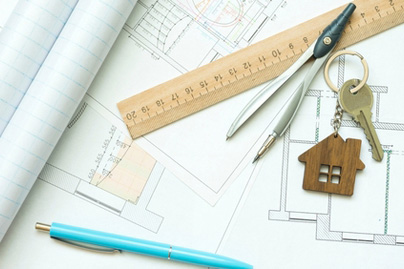Thank you!
Our representative will contact you shortly
Error occurred while submitting data. Please try again after some time.
Fill in the details below

We will call you back as soon as possible

# Square Feet to Gaj

Jan 02, 2023It is easy to understand the relationship between Square Feet and Gaj. The importance of a sq ft to gaj comes into the picture when you are looking forward to buying a house or land. Knowing explicit details about the measurements is crucial when buying a plot or land.

Understanding the conversion of square feet to gaj and vice versa becomes a mandate if you have laid out an extensive plan for buying your own house. This knowledge will be of use to get a wide idea about the space area you will be spending your money on. This article will help you gather all the details about square feet to gaj conversions, definitions, and calculations.

## What Is a Square Foot?

AThe area covered in a single square is called a square foot. The four sides of the square are equivalent to 1 foot. There are many ways to denote square feet, which are addressed by sq. ft or ft2.

Every city in India uses this as a standard measurement unit for conducting measurements. In addition to India, Canada, the US, the UK, Nepal, etc., other countries commonly use square feet for all their measurement activities. Square feet is an imperial unit and falls under the system of measurement. Measurement through square feet has gained traction globally, and this metric system is used everywhere, such as in flats, houses, and apartments.

Architects use square feet metrics primarily because it is easier to measure. In addition to that, it is also a convenient choice when measuring large square units. Nowadays, many machines and devices help to measure property dimensions, but inaccuracy can sometimes become a problem. Thus, the calculation will become very easy when you know the metrics better.

## What is a Gaj?

Gaj is a traditional land measurement unit commonly used in India and originated during the Mughal empire. Gaj unit is frequently used in the north across Delhi, Haryana, and Punjab regions.

Other cities like Bangladesh and Nepal are familiar with the Gaj term, which is used as Guz. It is also commonly known as a square yard. In addition to land measurements, it is also used for measuring objects and textiles. 9 square feet of the land area combine to form a Gaj, and in regards to square meters, the value of Gaj equals 0.82. In relation to measuring the length of the textile, 1 Gaj in square feet will be equal to 8.91.

## Converting Square Feet to Gaj

You can opt for square feet to gaj converter to have error-free results. This can be handled within seconds. However, it is best to know how to convert square feet into gaj.

When you know the conversion formula well, you can be certain about not making any mistakes. Say you are calculating sq ft. into gaj where the dimension of the area is 30 ft * 30 ft, which makes a total of 900 square feet.

To convert square feet to gaj, where we know 1 square ft. is equivalent to 0.1111 Gaj, all we need to do is multiply the square feet with the value of Gaj, i.e., 0.1111.

Calculation:

1 Square Feet = 0.1111 Gaj

∴ 30 sq. ft. = 30 * 0.1111 = 3.333 Gaj

Therefore,

200 sq. ft = 200 * 0.1111 = 22.22 Gaj

600 sq. ft = 600 * 0.1111 = 66.66 Gaj

## Difference Between Square Feet and Gaj

Square feet and Gaj are both used for land measurement. There is a difference between the two.

### Square Feet

Meaning

The use of square feet is regarded as the imperial unit, mostly used for land measurement.

Symbol

Square feet are denoted in sq. ft.

Conversion

Gaj equals sq. ft multiplied by 0.111.

Use

The use of square feet is mainly seen in real estate and architecture.

Relationship

1 square feet is equivalent to 0.112 Gaj.

### Gaj

Meaning

Gaj is a unit of length mainly used to describe the yards in various measures.

Symbol

Gaj is denoted by Guz or Gaz.

Conversion

Gaj equals 9 * square feet.

Use

It is used for the measurement of land and textiles.

Relationship

1 Gaj to square feet is equivalent to 9 square feet.

### Conclusion

After going through this article, you can make decisions about real-estate measurements. Using simple conversion methods can assist in understanding the greater differences between square feet, gaj, and other units.

Understanding the differences better will be of great use, especially when buying a property. With Fullerton Grihashakti, you can apply for a home loan that ranges up to INR 5 Crores*. With flexible repayment tenure and affordable interest rates, you can opt for a loan by understanding the dynamics of the kind of house you need. Interest rates start from only 9.50%* and tenures go up to 30 years. Check out our online eligibility calculator and get started today.

*Terms and Conditions apply. Loans are disbursed at the discretion of Fullerton Grihashakti.